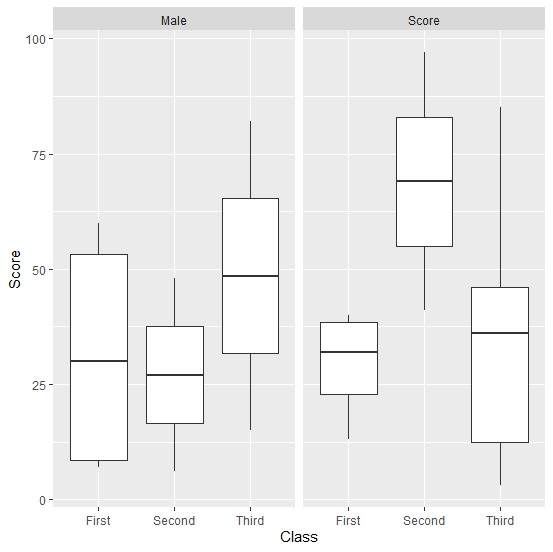# How to create boxplots based on two factor data in R?

To create boxplots based on two factor data, we can create facets for one of the factors, where each facet will contain the boxplots for the second factor.

For example, if we have a data frame called df that contains two factor columns say F1 and F2 and one numerical column say Num then the boxplot based on these two factors can be created by using the below given command −

ggplot(df,aes(F1,Num))+geom_boxplot()+facet_wrap(~F2)

## Example

Following snippet creates a sample data frame −

Gender<-sample(c("Male","Score"),20,replace=TRUE)
Class<-sample(c("First","Second","Third"),20,replace=TRUE)
Score<-sample(1:100,20)
df<-data.frame(Gender,Class,Score)
df

## Output

The following dataframe is created −

   Gender Class    Score
1  Score   First   26
2  Score   Second  97
3  Male    Third   15
4  Male    Second   6
5  Score   First   40
6  Score   Third   49
7  Male    First   60
8  Score   Second  41
9  Male    Third   82
10 Male    First    7
11 Score   Third   85
12 Male    Second  48
13 Score   Third   35
14 Score   First   38
15 Score   Third    3
16 Male    First   51
17 Score   Third   37
18 Male    First    9
19 Score   Third    5
20 Score   First   13

To load ggplot2 package and create boxplots for data in df, add the following code to the above snippet −

library(ggplot2)
ggplot(df,aes(Class,Score))+geom_boxplot()+facet_wrap(~Gender)

## Output

If you execute all the above given snippets as a single program, it generates the following Output −